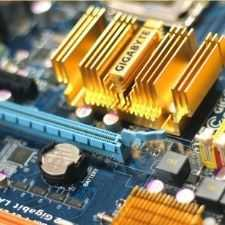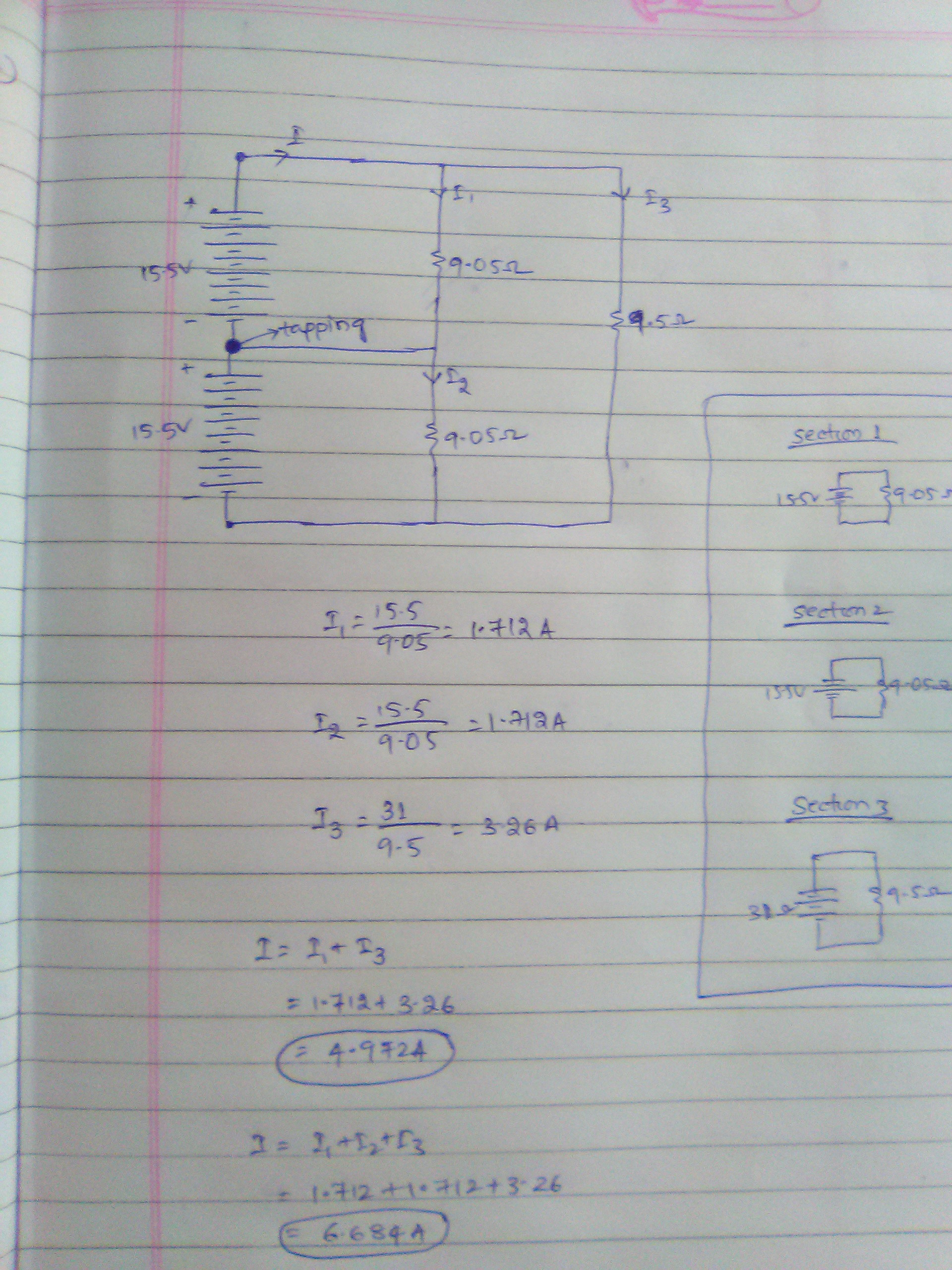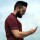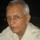Electronics
Community of Electronics Engineers to discuss all concepts, topics, questions and doubts.
504 Members
Join this group to post and comment.# What is the total current in the circuit?Is it 4.9A or 6.6A ?

There are three sections 1-2, 2-3, 1-3 and tapping is done in series.

What is the total current ?Mohit Patil • Feb 22, 2019can you explainKaustubh Katdare • Feb 22, 2019

@Vontari Keerthana - Better approach would be to explain how you arrived at two different answers. Could you give it a try?As they are in series I1 and I3 adds.

But as there is tapping im a little bit confused.

So can you help me out of it.@Kaustubh Katdare  please suggest what is the correct answer and complete solution.

I have got 4.9A, but my friend is saying that it may be 6.6A.@Vontari Keerthana , you were right.  The tapping at the middle two resistors joining the middle of the two battery banks can be ignored because there is no potential difference between the two ends of the wire.

Therefore, the total current will be the sum of the currents in the two parallel lines.

The current through the two resistors in series will be 31/(9.05+9.05)=1.713 A, the current in the other line is 31/9.5=3.26 A totaling 4.976 A.Thank you sir @Ramani Aswath